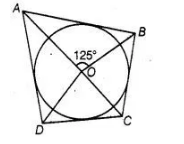# In figure, if ∠AOB = 125°, then ∠COD is equal to

Question:

In figure, if ∠AOB = 125°, then ∠COD is equal to(a) 62.5°

(b) 45°

(c) 35°

(d) 55°

Solution:

(d) We know that, the opposite sides of a quadrilateral circumscribing a circle subtend supplementary angles at the centre of the circle.

i.e., $\quad \angle A O B+\angle C O D=180^{\circ}$

$\Rightarrow \quad \angle C O D=180^{\circ}-\angle A O B$

$=180^{\circ}-125^{\circ}=55^{\circ}$